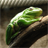# Count of fields in a row that satisfy a query

4104
8
01-27-2017 02:50 PMOccasional Contributor

I am wanting to calculate the count of fields in a row where designated fields in a feature class attribute table satisfy a query (in this case, where the value is greater than 0) and then write the count (sum) of the number of fields that satisfy this query into a new field.

This seems simple, but I am having a hard time wrapping my head around how to do this using SQL or Python. Any suggestions or advice would be much appreciated.

Tags (4)
1 Solution

Accepted SolutionsbyMVP Regular Contributor

You could use the field calculator and something like:

``````def FieldCount(fld1,fld2, ...):
fields = [fld1, fld2, ...] # field values to list
return sum(f > 0 for f in fields)

FieldCount( !F01!, !F02!, ... )‍‍‍‍‍‍‍‍‍‍‍‍‍‍‍‍‍‍‍‍‍‍‍‍‍‍‍‍‍‍‍‍‍‍‍``````

Or this for the function:

``````def FieldCount(fld1,fld2, ...):
return sum(v > 0 for k, v in locals().iteritems())‍‍‍‍‍‍‍‍‍‍‍‍‍‍‍‍‍‍‍‍‍‍‍‍‍‍‍‍‍‍‍‍``````

8 RepliesFrequent Contributor

Have you looked at using cursors to pull the values of each value of each field you need in a row to check see if it is greater than 0?

https://pro.arcgis.com/en/pro-app/arcpy/data-access/updatecursor-class.htmbyMVP Regular Contributor

In Python, Joshua Bixby‌ covers the options in this blog post.

In SQL, you can do something like

``select count(*) from TableName where FieldName > 0‍‍``

EDIT:

Just to clarify your question, I will edit it for what I think you meant to say...

I am wanting to calculate the count of rows in a field where designated fields in a feature class attribute table satisfy a query (in this case, where the value is greater than 0) and then write the count (sum) of the number of rows that satisfy this query into a new field.Frequent ContributorOccasional Contributor

Nope, I meant the count of fields per row (per feature) where designated fields satisfy a query. Here I am looking for the the count of fields (this would only pertain to 5 of the 40 fields in the feature class) which have a value greater than zero. I would also then like to write the count (sum) of the number of fields that satisfy this query into a new field, so this new field would have a count of 0 (or NULL) or an integer from 1 to 5.

Does this help? I apologize for the lack of clarity. Thanks for the help! I would also like to eventually integrate this into a Python script (eventually).byMVP Regular Contributor

Some sample data would help. I think I understand but it is a very unusual request and I want to make sure.Occasional Contributor

I  was looking to get the sum of the number of particular fields in a row that had a value greater than zero (to find how many of these unique attributes each feature had, e.g. ). I think Randy hit on exactly what I was looking for. Thanks a bunch!byMVP Regular Contributor

You could use the field calculator and something like:

``````def FieldCount(fld1,fld2, ...):
fields = [fld1, fld2, ...] # field values to list
return sum(f > 0 for f in fields)

FieldCount( !F01!, !F02!, ... )‍‍‍‍‍‍‍‍‍‍‍‍‍‍‍‍‍‍‍‍‍‍‍‍‍‍‍‍‍‍‍‍‍‍‍``````

Or this for the function:

``````def FieldCount(fld1,fld2, ...):
return sum(v > 0 for k, v in locals().iteritems())‍‍‍‍‍‍‍‍‍‍‍‍‍‍‍‍‍‍‍‍‍‍‍‍‍‍‍‍‍‍‍‍``````